# Problems on Trains - Quantitative Aptitude (MCQ) questions

Dear Readers, Welcome to Quantitative Aptitude Problems on Trains questions and answers with explanation. These Problems on Trains solved examples with shortcuts and tricks will help you learn and practice for your Placement Test and competitive exams like Bank PO, IBPS PO, SBI PO, RRB PO, RBI Assistant, LIC,SSC, MBA - MAT, XAT, CAT, NMAT, UPSC, NET etc.

After practicing these tricky Problems on Trains multiple choice questions, you will be exam ready to deal with any objective type questions.

1)   Jammu Express is 1.2 km long and is running along the Kerala express at speed of 80km/hr. Kerala express is 2/3rd the length of Jammu express and runs at 60 km/hr. How much time will it take for Jammu express to overtake the Kerala express?
- Published on 11 Apr 17

a. 12 minutes
b. 6 minutes
c. 3 minutes
d. 8 minutes
 Answer  Explanation ANSWER: 6 minutes Explanation: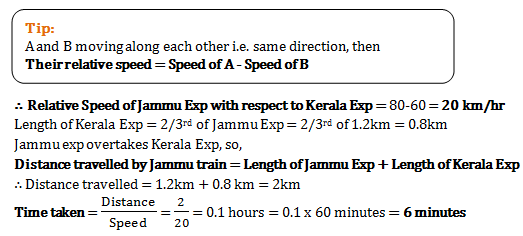2)   If a train crosses a pole in 12 seconds while travelling at a speed of 45km/hr, then in how much time will the train cross a bridge of length 600m at same speed?
- Published on 11 Apr 17

a. 1 minute 20 seconds
b. 60 seconds
c. 40 seconds
d. 45 seconds
 Answer  Explanation ANSWER: 60 seconds Explanation: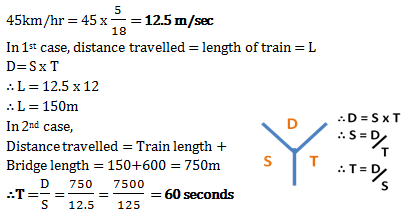3)   A bus is going to Goa from Pune. With 4 stops of 15 minute each, the average speed of the bus comes out to be 45 m/hr. But if the driver takes the bus without any stops, the average peed comes out to be 50 km/hr.  How far is Goa from Pune?
- Published on 11 Apr 17

a. 1350 km
b. 450 km
c. 360 km
d. 630 km
 Answer  Explanation ANSWER: 450 km Explanation: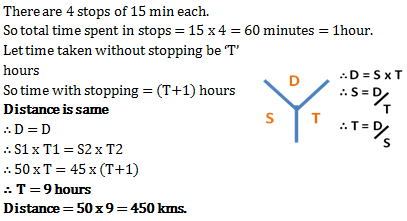4)   Consider statements A and B given below and decide whether they are enough to find the time needed for two trains to cross each other when they are travelling in opposite directions and one train is moving at a speed of 86 km/hr.

Statement A – Trains are of equal length of 350m
Statement B – Other train is moving with speed 83 km/hr

- Published on 11 Apr 17

a. Only statement B is enough
b. Any one statement if given is enough to find the answer
c. Both statements together are needed to find the answer
d. Even both statements together are not enough to find the answer
 Answer  Explanation ANSWER: Both statements together are needed to find the answer Explanation: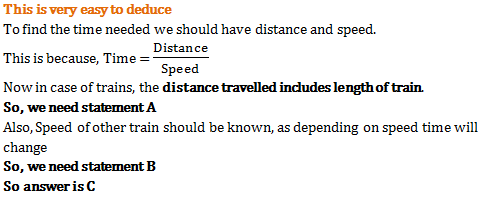5)   A train passes a telegraph post in 8 seconds and a 264 m long bridge in 20 seconds. What is the length of the train
- Published on 07 Jul 17

a. 180 m
b. 176 m
c. 164 m
d. 158 m
 Answer  Explanation ANSWER: 176 m Explanation: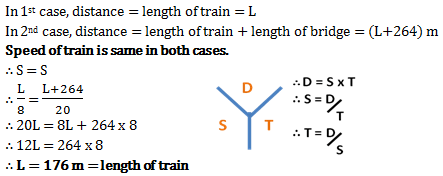6)   A train 120 m long passes a telegraph post in 6 seconds. Find the speed of the train.
- Published on 07 Jul 17

a. 60 km/hr
b. 72.5 km/hr
c. 80 km/hr
d. 72 km/hr
 Answer  Explanation ANSWER: 72 km/hr Explanation: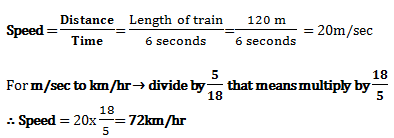7)   A train moves with the speed of 180 km/hr. Its speed (in metres per second) is
- Published on 07 Jul 17

a. 5
b. 40
c. 30
d. 50
 Answer  Explanation ANSWER: 50 Explanation: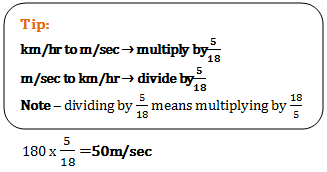8)   From P and Q, two trains start moving towards each other at the same time. Their speeds are 120 km/hr and 100 km/hr, respectively. When the two trains meet each other, one train has covered 40 km more than other train. Find the distance between P and Q?
- Published on 17 Mar 17

a. 180 kms
b. 260 kms
c. 220 kms
d. 440 kms
 Answer  Explanation ANSWER: 440 kms Explanation: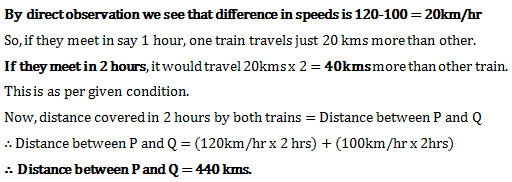9)   There are 2 trains P and Q moving in same direction. They are of equal length and cross a stationary pole in 5 seconds and 6 seconds respectively. In how much time would they cross each other?
- Published on 15 Mar 17

a. 30 seconds
b. 11 seconds
c. 60 seconds
d. 1 second
 Answer  Explanation ANSWER: 60 seconds Explanation: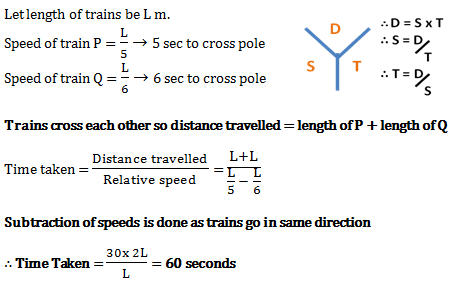10)   A train having length 150 m passes a platform of 550 m length. The time taken for it is 56 seconds. In how much time will this train take to pass the platform of 250 m length?
- Published on 15 Mar 17

a. 32 seconds
b. 24 seconds
c. 28 seconds
d. 30 seconds
 Answer  Explanation ANSWER: 32 seconds Explanation: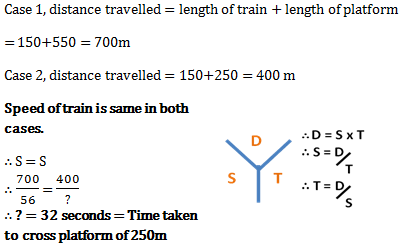1 2 3 4